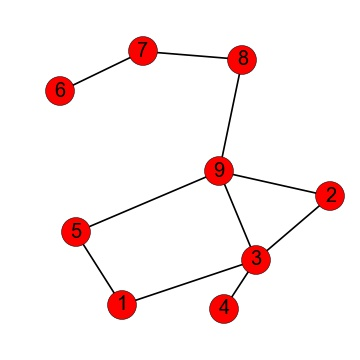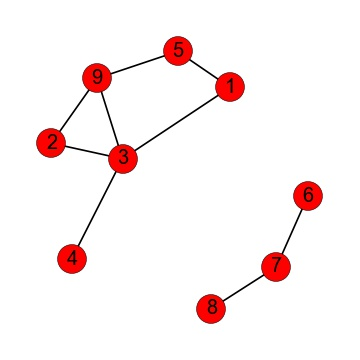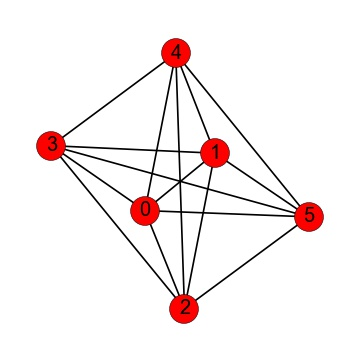Probability

# Tracing PathsIn the graph above, is it possible to trace a path from node 4 to node 6?In the graph above, is is possible to trace a path from node 5 to node 4 that uses 3 or fewer edges?How many paths are there between node 4 and node 8 where each path is at most of length 4 (in other words, at most 4 edges long)?How many distinct paths of length 4 (paths using 4 edges) that do not visit the same node more than once are there between node 0 and node 1?How many paths are there between node number 9 and node number 7?

×